This book is archived and will be removed July 6, 2022. Please use the updated version.

Atomic Structure

# 58 Orbital Magnetic Dipole Moment of the Electron

### Learning Objectives

By the end of this section, you will be able to:

• Explain why the hydrogen atom has magnetic properties
• Explain why the energy levels of a hydrogen atom associated with orbital angular momentum are split by an external magnetic field
• Use quantum numbers to calculate the magnitude and direction of the orbital magnetic dipole moment of a hydrogen atom

In Bohr’s model of the hydrogen atom, the electron moves in a circular orbit around the proton. The electron passes by a particular point on the loop in a certain time, so we can calculate a current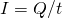. An electron that orbits a proton in a hydrogen atom is therefore analogous to current flowing through a circular wire ((Figure)). In the study of magnetism, we saw that a current-carrying wire produces magnetic fields. It is therefore reasonable to conclude that the hydrogen atom produces a magnetic field and interacts with other magnetic fields.

(a) Current flowing through a circular wire is analogous to (b) an electron that orbits a proton in a hydrogen atom.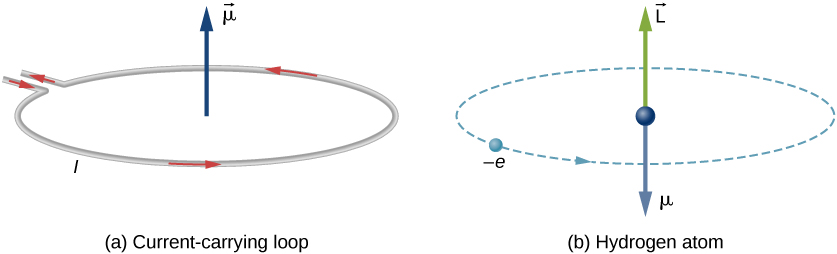The orbital magnetic dipole moment is a measure of the strength of the magnetic field produced by the orbital angular momentum of an electron. From Force and Torque on a Current Loop, the magnitude of the orbital magnetic dipole moment for a current loop is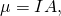where I is the current and A is the area of the loop. (For brevity, we refer to this as the magnetic moment.) The current I associated with an electron in orbit about a proton in a hydrogen atom is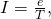where e is the magnitude of the electron charge and T is its orbital period. If we assume that the electron travels in a perfectly circular orbit, the orbital period is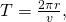where r is the radius of the orbit and v is the speed of the electron in its orbit. Given that the area of a circle is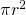, the absolute magnetic moment is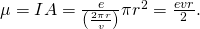It is helpful to express the magnetic momentum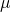in terms of the orbital angular momentum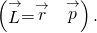Because the electron orbits in a circle, the position vector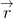and the momentum vector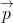form a right angle. Thus, the magnitude of the orbital angular momentum is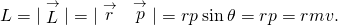Combining these two equations, we have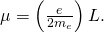In full vector form, this expression is written as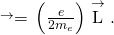The negative sign appears because the electron has a negative charge. Notice that the direction of the magnetic moment of the electron is antiparallel to the orbital angular momentum, as shown in (Figure)(b). In the Bohr model of the atom, the relationship between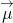and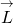in (Figure) is independent of the radius of the orbit.

The magnetic momentcan also be expressed in terms of the orbital angular quantum number l. Combining (Figure) and (Figure), the magnitude of the magnetic moment is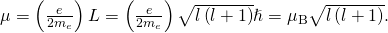The z-component of the magnetic moment is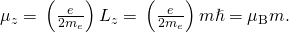The quantity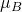is a fundamental unit of magnetism called the Bohr magneton, which has the value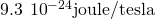(J/T) orQuantization of the magnetic moment is the result of quantization of the orbital angular momentum.

As we will see in the next section, the total magnetic dipole moment of the hydrogen atom is due to both the orbital motion of the electron and its intrinsic spin. For now, we ignore the effect of electron spin.

Orbital Magnetic Dipole Moment What is the magnitude of the orbital dipole magnetic momentof an electron in the hydrogen atom in the (a) s state, (b) p state, and (c) d state? (Assume that the spin of the electron is zero.)

Strategy The magnetic momentum of the electron is related to its orbital angular momentum L. For the hydrogen atom, this quantity is related to the orbital angular quantum number l. The states are given in spectroscopic notation, which relates a letter (s, p, d, etc.) to a quantum number.

Solution The magnitude of the magnetic moment is given in (Figure):1. For the s state,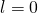so we have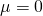and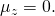2. For the p state,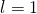and we have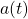3. For the d state,and we obtain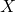Significance In the s state, there is no orbital angular momentum and therefore no magnetic moment. This does not mean that the electron is at rest, just that the overall motion of the electron does not produce a magnetic field. In the p state, the electron has a magnetic moment with three possible values for the z-component of this magnetic moment; this means that magnetic moment can point in three different polar directions—each antiparallel to the orbital angular momentum vector. In the d state, the electron has a magnetic moment with five possible values for the z-component of this magnetic moment. In this case, the magnetic moment can point in five different polar directions.

A hydrogen atom has a magnetic field, so we expect the hydrogen atom to interact with an external magnetic field—such as the push and pull between two bar magnets. From Force and Torque on a Current Loop, we know that when a current loop interacts with an external magnetic field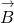, it experiences a torque given by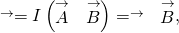where I is the current,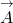is the area of the loop,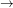is the magnetic moment, andis the external magnetic field. This torque acts to rotate the magnetic moment vector of the hydrogen atom to align with the external magnetic field. Because mechanical work is done by the external magnetic field on the hydrogen atom, we can talk about energy transformations in the atom. The potential energy of the hydrogen atom associated with this magnetic interaction is given by (Figure):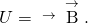If the magnetic moment is antiparallel to the external magnetic field, the potential energy is large, but if the magnetic moment is parallel to the field, the potential energy is small. Work done on the hydrogen atom to rotate the atom’s magnetic moment vector in the direction of the external magnetic field is therefore associated with a drop in potential energy. The energy of the system is conserved, however, because a drop in potential energy produces radiation (the emission of a photon). These energy transitions are quantized because the magnetic moment can point in only certain directions.

If the external magnetic field points in the positive z-direction, the potential energy associated with the orbital magnetic dipole moment is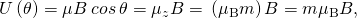whereis the Bohr magneton and m is the angular momentum projection quantum number (or magnetic orbital quantum number), which has the values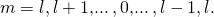For example, in theelectron state, the total energy of the electron is split into three distinct energy levels corresponding to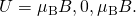The splitting of energy levels by an external magnetic field is called the Zeeman effect. Ignoring the effects of electron spin, transitions from thestate to a common lower energy state produce three closely spaced spectral lines ((Figure), left column). Likewise, transitions from thestate produce five closely spaced spectral lines (right column). The separation of these lines is proportional to the strength of the external magnetic field. This effect has many applications. For example, the splitting of lines in the hydrogen spectrum of the Sun is used to determine the strength of the Sun’s magnetic field. Many such magnetic field measurements can be used to make a map of the magnetic activity at the Sun’s surface called a magnetogram ((Figure)).

The Zeeman effect refers to the splitting of spectral lines by an external magnetic field. In the left column, the energy splitting occurs due to transitions from the state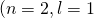) to a lower energy state; and in the right column, energy splitting occurs due to transitions from the state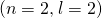to a lower-energy state. The separation of these lines is proportional to the strength of the external magnetic field.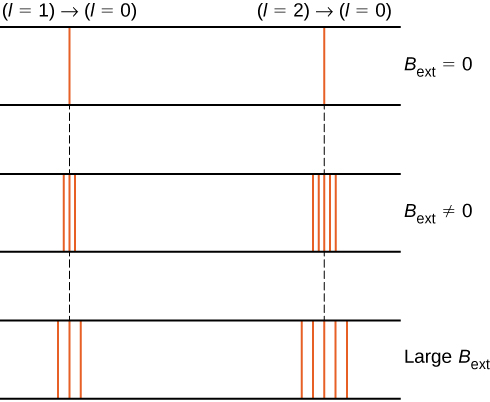A magnetogram of the Sun. The bright and dark spots show significant magnetic activity at the surface of the Sun. (credit: NASA, SDO)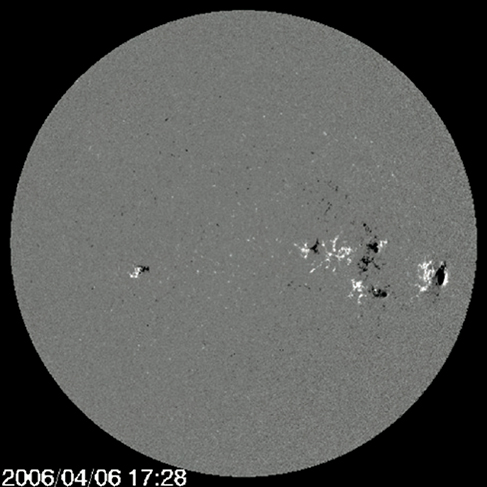### Summary

• A hydrogen atom has magnetic properties because the motion of the electron acts as a current loop.
• The energy levels of a hydrogen atom associated with orbital angular momentum are split by an external magnetic field because the orbital angular magnetic moment interacts with the field.
• The quantum numbers of an electron in a hydrogen atom can be used to calculate the magnitude and direction of the orbital magnetic dipole moment of the atom.

### Conceptual Questions

Explain why spectral lines of the hydrogen atom are split by an external magnetic field. What determines the number and spacing of these lines?

A hydrogen atom is placed in a magnetic field. Which of the following quantities are affected? (a) total energy; (b) angular momentum; (c) z-component of angular momentum; (d) polar angle.

a, c, d; The total energy is changed (Zeeman splitting). The work done on the hydrogen atom rotates the atom, so the z-component of angular momentum and polar angle are affected. However, the angular momentum is not affected.

On what factors does the orbital magnetic dipole moment of an electron depend?

### Problems

Find the magnitude of the orbital magnetic dipole moment of the electron in in the 3p state. (Express your answer in terms of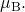)

The 3p state corresponds to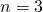,. Therefore,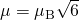A current of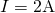flows through a square-shaped wire with 2-cm side lengths. What is the magnetic moment of the wire?

Estimate the ratio of the electron magnetic moment to the muon magnetic moment for the same state of orbital angular momentum. (Hint: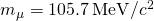The ratio of their masses is 1/207, so the ratio of their magnetic moments is 207. The electron’s magnetic moment is more than 200 times larger than the muon.

Find the magnitude of the orbital magnetic dipole moment of the electron in in the 4d state. (Express your answer in terms of)

For a 3d electron in an external magnetic field of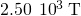, find (a) the current associated with the orbital angular momentum, and (b) the maximum torque.

a. The 3d state corresponds to,. So,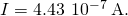b. The maximum torque occurs when the magnetic moment and external magnetic field vectors are at right angles (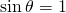. In this case: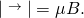An electron in a hydrogen atom is in the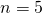,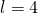state. Find the smallest angle the magnetic moment makes with the z-axis. (Express your answer in terms of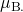)

Find the minimum torque magnitude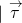| that acts on the orbital magnetic dipole of a 3p electron in an external magnetic field of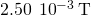.

A 3p electron is in the stateand. The minimum torque magnitude occurs when the magnetic moment and external magnetic field vectors are most parallel (antiparallel). This occurs when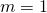.The torque magnitude is given by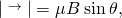Where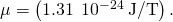For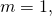we have: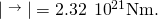An electron in a hydrogen atom is in 3p state. Find the smallest angle the magnetic moment makes with the z-axis. (Express your answer in terms of)

Show that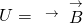.

(Hint: An infinitesimal amount of work is done to align the magnetic moment with the external field. This work rotates the magnetic moment vector through an angle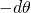(toward the positive z-direction), where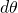is a positive angle change.)

An infinitesimal work dW done by a magnetic torque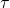to rotate the magnetic moment through an angle: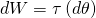,
where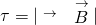. Work done is interpreted as a drop in potential energy U, so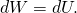The total energy change is determined by summing over infinitesimal changes in the potential energy: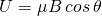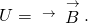### Glossary

Bohr magneton
magnetic moment of an electron, equal to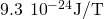or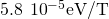magnetic orbital quantum number
another term for the angular momentum projection quantum number
magnetogram
pictoral representation, or map, of the magnetic activity at the Sun’s surface
orbital magnetic dipole moment
measure of the strength of the magnetic field produced by the orbital angular momentum of the electron
Zeeman effect
splitting of energy levels by an external magnetic field